#[Rust] 程式設計教學：控制結構 (Control Structure) 或控制流程 (Control Flow)

## 前言

• 選擇結構 (selection structure) 或條件敘述 (conditional)
• 迭代結構 (iteration structure) 或迴圈 (loop)

## 條件敘述 (Conditional)

### `if`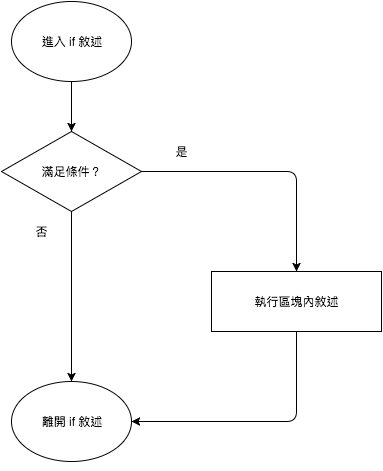`if` 寫成虛擬碼如下：

``````if condition {
statement
}``````

``````fn main() {
let x = 3;

if x > 0 {
println!("x is larger than zero");
}
}``````

`if` 還可以選擇性加入 `else` 區塊，這時候變成二元敘述。其想法如以下流程圖：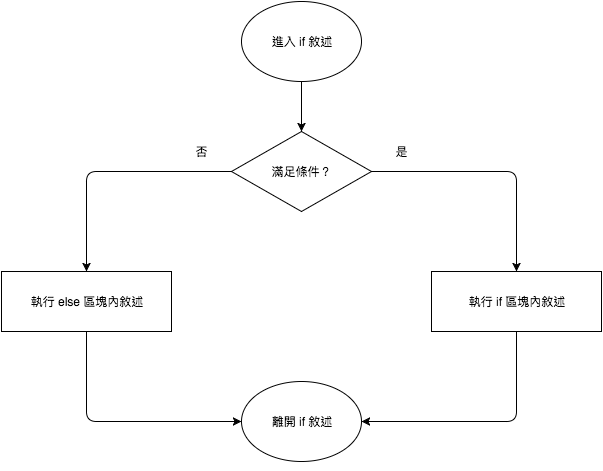``````if condition {
statement 1
} else {
statement 2
}``````

``````fn main() {
let x = 3;

if x > 10 {
println!("x is larger than 10");
} else {
println!("x is not larger than 10");
}
}``````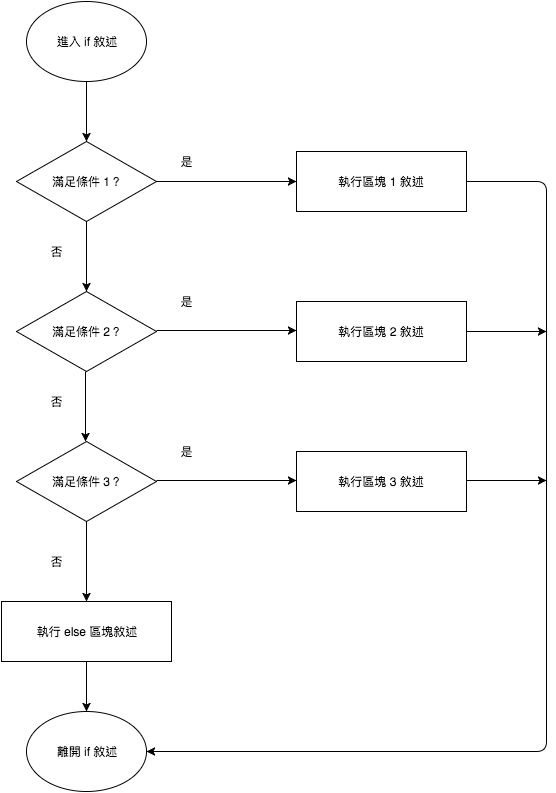``````if condition 1 {
statement 1
} else if condition 2 {
statement 2
} else {
statement 3
}``````

``````fn main() {
let x = 0;

if x > 0 {
println!("x is larger than zero");
} else if x == 0 {
println!("x is equal to zero");
} else {  // x < 0
println!("x is smaller than zero");
}
}``````

### `if` 區塊是表達式而非敘述

`if` 也可以作為回傳值，如下例：

``````fn main() {
let score = 83;

let grade = if score > 90 {
"A"
} else if score > 80 {
"B"
} else if score > 70 {
"C"
} else if score > 60 {
"D"
} else {
"F"
};

}``````

### `match`

``````fn main() {

"A" => println!("Wonderful"),
"B" => println!("Good"),
"C" => println!("So so"),
"D" => println!("It could be better"),
"F" => println!("Oh, oh"),
_ => println!("Unknown grade"),  // Error on removal.
}
}``````

``````fn main() {

"A" => println!("Wonderful"),
"B" => println!("Good"),
"C" => println!("So so"),
"D" => println!("It could be better"),
"F" => println!("Oh, oh"),
}
}``````

``error[E0004]: non-exhaustive patterns: `&_` not covered``

`match` 要窮舉所有情境的設計是安全上的考量，以免程式設計者遺漏一些未考慮的情境。這是 Rust 在安全上的考量。

### `match` 是表達式而非敘述

`match``if` 相同，也可以做為回傳值。如下例：

``````fn main() {
let char = 'c';

let sound = match char {
'A' | 'a' | 'E' | 'e' | 'I' | 'i' | 'O' | 'o' | 'U' |'u' => "vowel",
'A' ... 'Z' | 'a' ... 'z' => "consonant",
_ => "other"
};

assert_eq!(sound, "consonant");
}``````

## 迴圈 (Loop)

### `loop`

`loop` 是最簡單的一種迴圈，其想法如下：``````loop {
statement
}``````

``````fn main() {
loop {
println!("Hello, World");
}
}``````

### `while`

`while` 在程式滿足終止條件前，會不間斷地執行該區塊內的程式碼。其想法如下：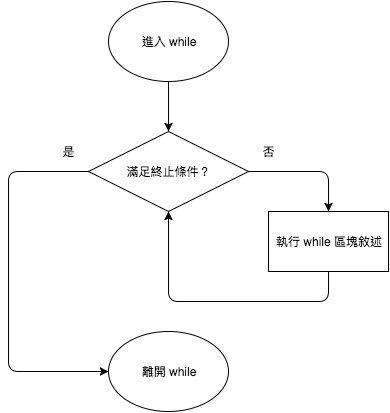``````while condition {
statement
}``````

``````fn main() {
let mut count = 10;

while count > 0 {
println!("Count down {}", count);
count -= 1;
}
}``````

``````fn main() {
while true {
println!("Hello, World");
}
}``````

### `for`

`for` 迴圈和 `while` 迴圈不同，`for` 會有明確的執行次數。Rust 的 `for` 迴圈的想法如下：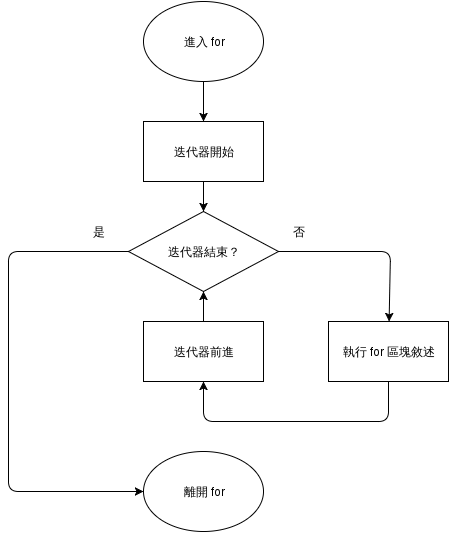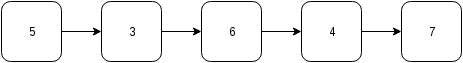``````for variable in iterator {
statement
}``````

``````fn main() {
// Iterate from 1 to 10
for i in 1..(10+1) {
println!("{}", i);
}
}``````

``````fn main() {
for _ in 1..(10+1) {
println!("Hello, World");
}
}``````

``````fn main() {
for i in (1..).step_by(2).take(5) {
println!("{}", i);
}
}``````

``````fn main() {
let mut i = 1;
while i < 10 {
println!("{}", i);
i += 2;
}
}``````

### 改變迴圈運作

``````fn main() {
let mut i = 1;
let mut flag = false;

while !flag {
println!("{}", i);
i += 1;

if i > 5 {
flag = true;
}
}
}``````

`break` 的作用是中斷迴圈，通常會寫在條件敘述內。以上的範例可以改寫如下：

``````fn main() {
let mut i = 1;

loop {
println!("{}", i);
i += 1;

if i > 5 {
break;
}
}
}``````

`continue` 不會中斷迴圈，但會跳過同一個迴圈內該指令之後的程式碼。範例如下：

``````fn main() {
for i in 1..11 {
if i % 2 == 0 {
continue;
}

println!("{}", i);
}
}``````

### 迴圈標籤

``````fn main() {
'outer: for x in 0..10 {
'inner: for y in 0..10 {
if x % 2 == 0 { continue 'outer; } // continues the loop over x
if y % 2 == 0 { continue 'inner; } // continues the loop over y
println!("x: {}, y: {}", x, y);
}
}
}``````

## (案例選讀) 終極密碼

``````Set the minimum and the maximum.

Set a random answer between the minimum and the maximum.

loop {

If the guess is correct, leave the loop.
}``````

``````[dependencies]
rand = "0.3"``````

``````// Call rand package for random number generation
extern crate rand;

use std::io;
use std::io::Write;
use rand::Rng;

fn main() {
// Set the limit of answer
const MAX: u32 = 100;
const MIN: u32 = 1;

// Set the limit of user input
let mut upper = MAX;
let mut lower = MIN;

// Get a random number between MIN and MAX

loop {
// Prompt for user input
print!("Input a number ({}-{}):", lower, upper);
// Flush standard out
let _ = io::stdout().flush();

let mut input = String::new();
io::stdin()

// Parse integer
let guess = match input.trim().parse::<u32>() {
Ok(n) => n,
Err(_) => {
println!("Not a valid number");
continue;
}
};

// Check the range of the guess
if guess < lower || guess > upper {
println!("The number should be between {} and {}",
lower, upper);
continue;
}

// Check whether the guess is correct